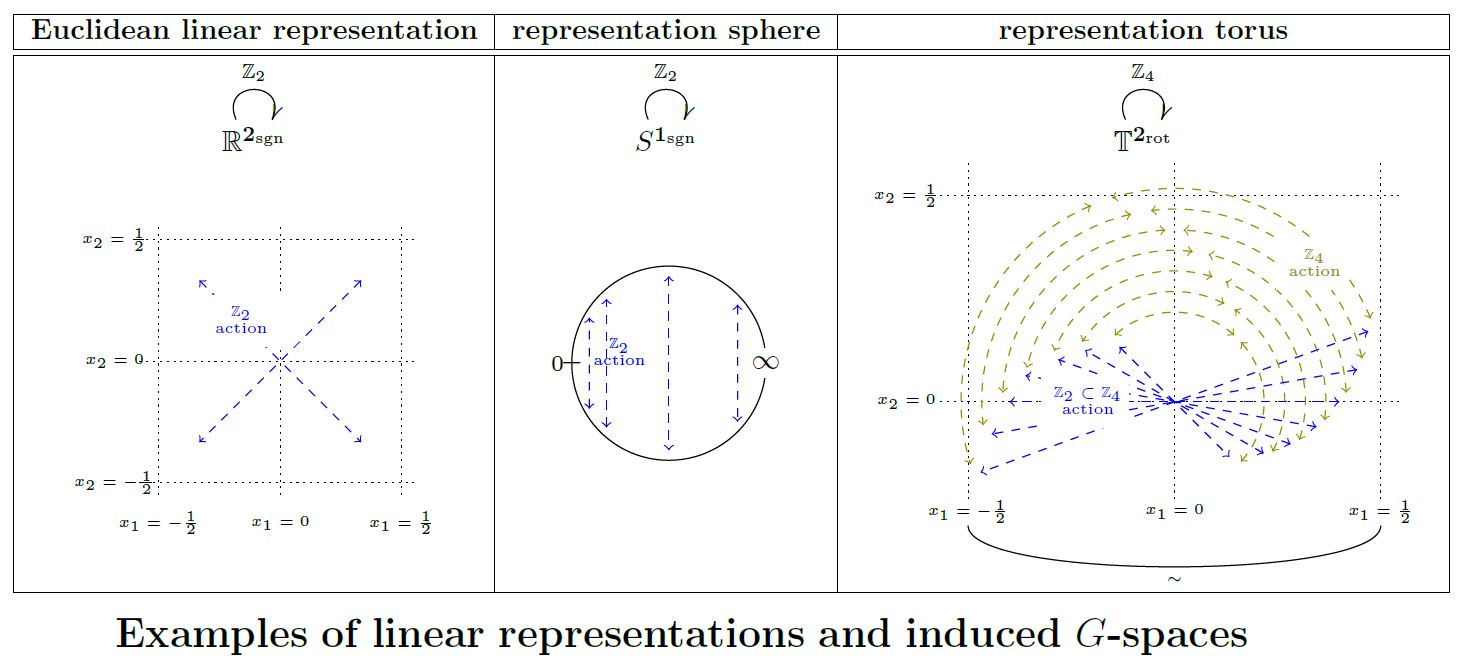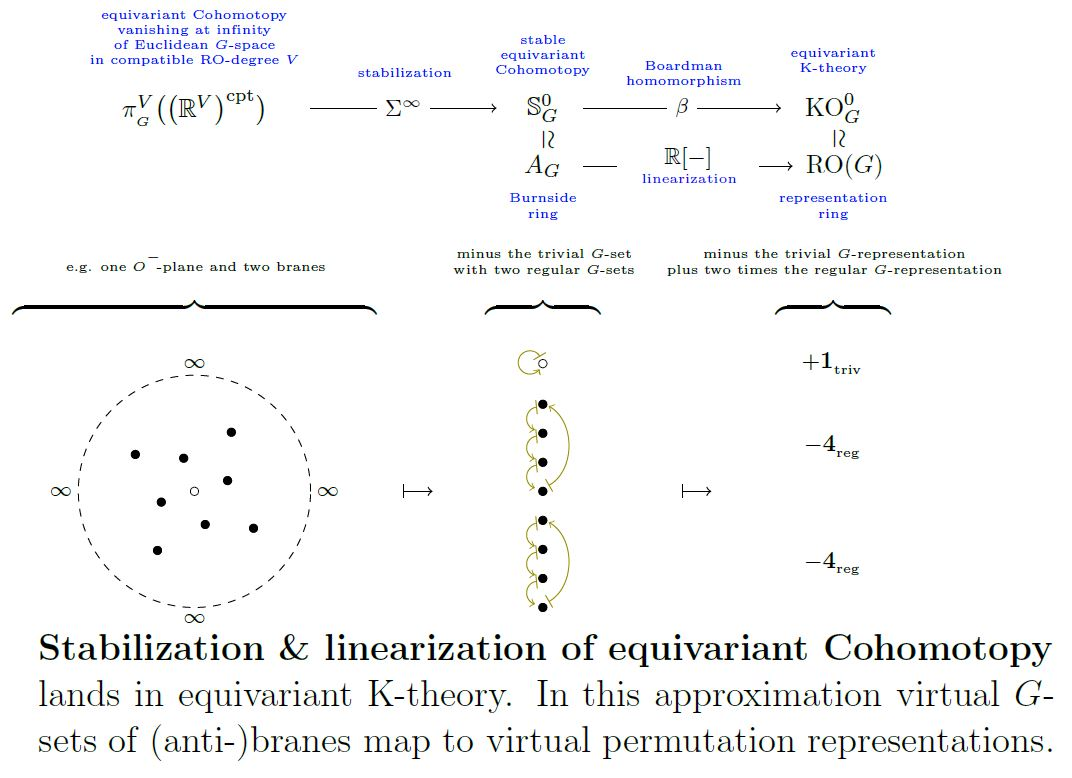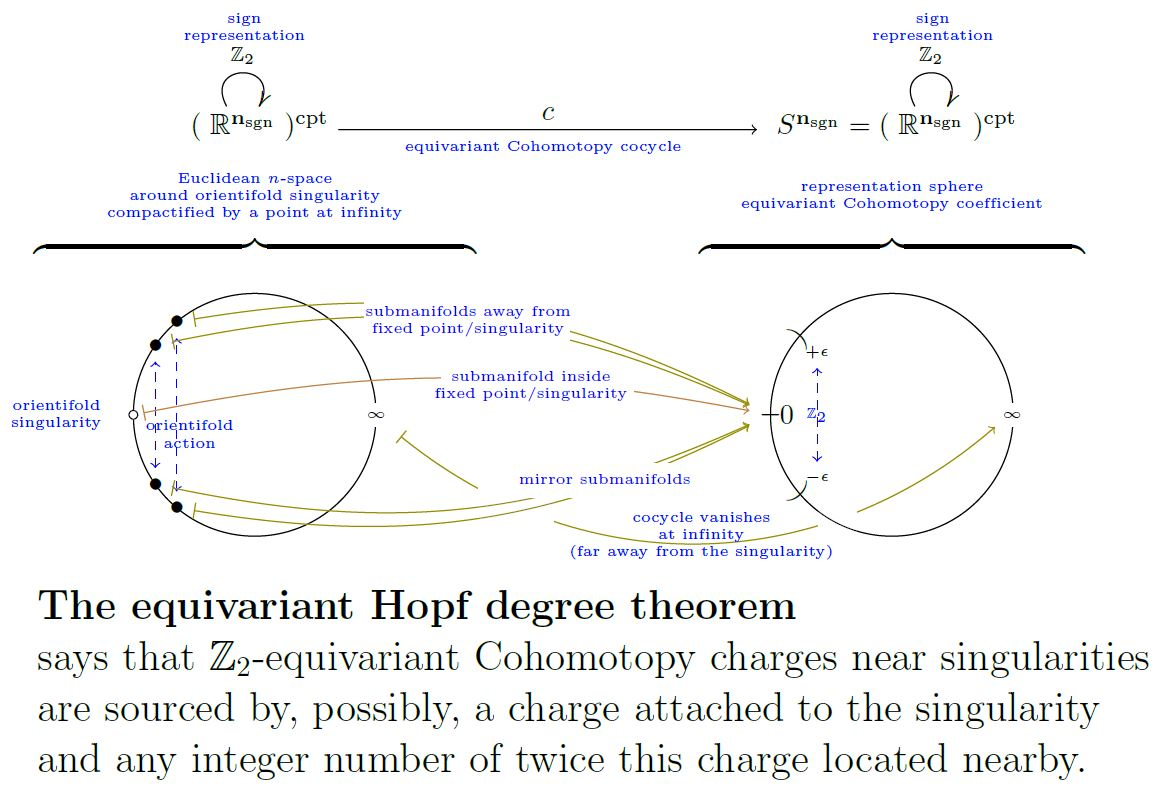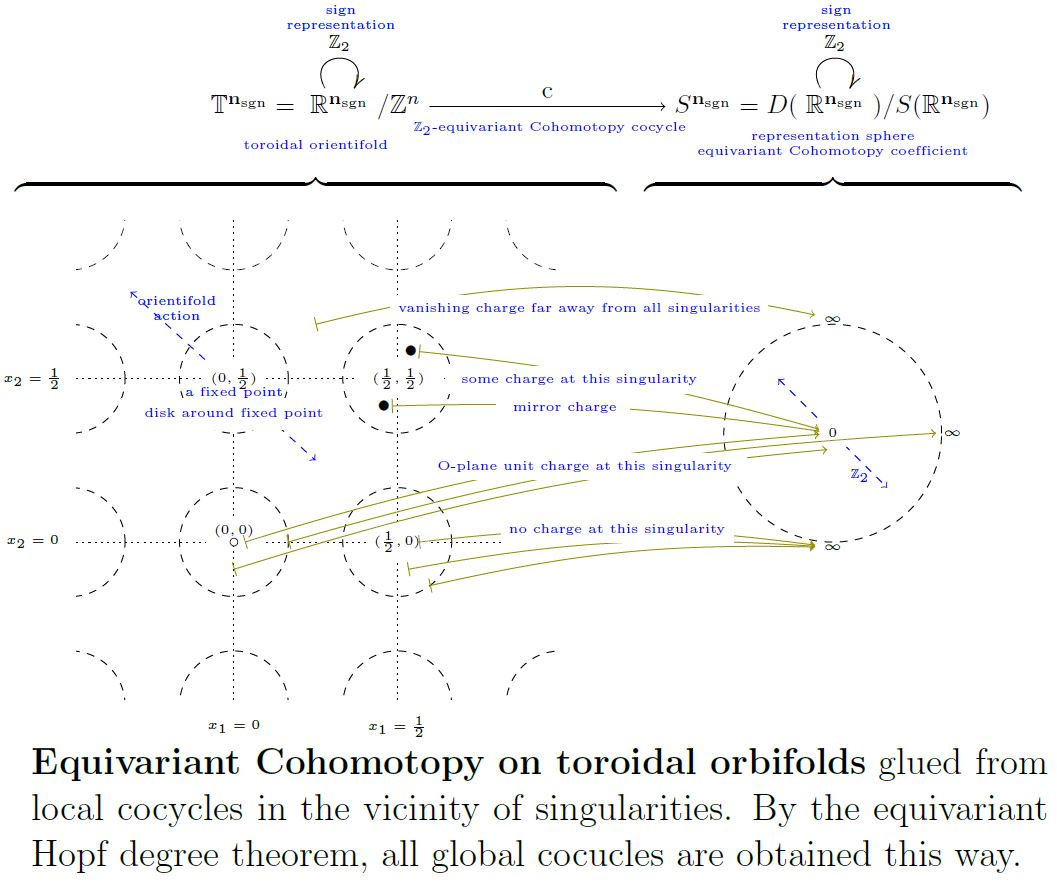# nLab equivariant Hopf degree theorem

Contents

### Context

#### Representation theory

representation theory

geometric representation theory

# Contents

## Idea

The equivariant Hopf degree theorem – Theorem below – generalizes the plain Hopf degree theorem from plain homotopy theory to equivariant homotopy theory, due to tomDieck 79, 8.4.

## Preliminaries: Matching $G$-Spaces

We need the following list of ingredients and assumptions:

Let $G$ be a finite group. For $H \subset G$ a subgroup, write

(1)$W_G H \coloneqq (N_G H) / H$

for its Weyl group.

For $X$ a G-space, we write

(2)$Isotr_X(G) \subset Sub(G)$

for the subset of the subgroup lattice on the isotropy groups of $X$, hence those subgroups which appear as stabilizer subgroups $Stab_G(x)$ of some point $x \in X$. This means that if $H_1, H_2 \in Iso_X(G)$ and $H_1 \lt H_2$ is a strict inclusion, then the fixed loci differ $X^{H_1} \gt X^{H_2}$.

###### Definition

(matching pair of $G$-spaces)

For $G$ a finite group, we say that a pair $X,Y \in G Spaces$ of topological G-spaces, where $X$ is a G-CW-complex, is a matching pair if the following conditions are satisfied for all isotropy groups $H \in Isotr_X(G)$ (2) of the $G$-action on $X$.

1. The fixed point space $X^H$ is a $W_G H = (N_G H) / H$-complex of finite dimension $dim\big( X^H\big) \in \mathbb{N}$;

2. $H^{dim(X^H)}\Big( X^H , \mathbb{Z}\Big) \simeq \mathbb{Z}$ (integral cohomology of the fixed point space),

this implies that the action of $W_G(H)$ on cohomology induces a group homomorphism

(3)$e_{H,X} \;\colon\; W_G(H) \longrightarrow Aut_{Ab}(\mathbb{Z}) \simeq \mathbb{Z}^\times$

to be called the orientation behaviour of the action of $W_G(H)$ on $X^H$;

and

1. $Y^H$ is $(dim(X^H)-1)$-connected

$\pi_{ \lt \mathrm{dim}\big( X^H \big) } \big( Y^H \big) \;=\; 0$

(hence connected if $dim\left(X^H\right) = 1$, simply connected if $dim\left(X^H\right) = 2$, etc.);

2. $\pi_{dim(X^H)}\big( Y^H\big) \simeq \mathbb{Z}$ (homotopy groups of fixed point space),

with the previous point this implies (by the Hurewicz theorem) that $H^{dim(X^H)}\Big( Y^H , \mathbb{Z}\Big) \simeq \mathbb{Z}$ and hence orientation behaviour (3) $e_{H,Y} \;\colon\; W_G(H) \to \mathbb{Z}^\times$

3. $e_{H,X} = e_{H,Y}$, the orientation behaviour (3) of $X$ and $Y$ agrees at all isotropy groups.

For simplicity we also demand that

• $dim(X^H) \geq 1$.

Given a matching pair of $G$-spaces, we say that a choice of generators in the cohomology groups of all the fixed strata

(4)\begin{aligned} or_{X,H} & = \pm 1 \in \mathbb{Z} \simeq H^{dim(X^H)}\big(X^H, \mathbb{Z} \big) \\ or_{Y,H} & = \pm 1 \in \mathbb{Z} \simeq H^{dim(X^H)}\big(Y^H, \mathbb{Z} \big) \end{aligned}

is a choice of singularity-wise orientations. Given such a choice and an equivariant continuous function $f \colon X \to Y$, we have for each isotropy group $H \in Isotr_X(G)$ that the continuous function $f^H \;\colon\; X^H \to Y^H$ has a well-defined integer degree

(5)$deg(f^H) \; \in \;\mathbb{Z} \,.$
###### Example

(representation spheres match representation spheres)

Let $G$ be a finite group and $V \in RO(G)$ a finite-dimensional orthogonal linear representation of $G$. Then the pair

$(X \coloneqq S^V,\; Y \coloneqq S^V)$

consisting of two copies of the representation sphere of $V$ is a matching pair of $G$-spaces, according to Def. .

###### Proof

First notice that an $H$-fixed locus of any $G$-representation sphere is itself a $W_G(H)$-representation sphere, since $\left(S^V\right)^H \simeq S^{\left( V^H\right)}$. That these are all WH-CW-complexes follows because generally G-representation spheres are G-CW-complexes. Moreover, since the topological spaces underlying all these fixed loci are n-spheres, and since we have the same spheres for $X$ and $Y$, the connectivity and orientability conditions in Def. are evidently satisfied.

###### Example

(representation tori match representation spheres)

Let $G$ be a finite group which arises as the point group $G \simeq S/N$ of a crystallographic group $S \subset Iso(E)$ of some Euclidean space $E$. Then the pair

$\big( X \coloneqq E/N \,; Y \coloneqq S^E \big)$

consisting of

1. the torus $E/N$ which is the quotient space of $E$ by the given crystallographic sub-lattice $N \subset E$ and equipped with the $G$-action descending from that on $E$ (this Prop.);

2. the representation sphere of the linear action of the point group $G$ on $E$

is a matching pair of $G$-spaces, according to Def. .

###### Proof

The torus $E/N$ carries the structure of a smooth manifold for which the $G$-action is smooth. Since $G$ is finite, also all its fixed loci $(E/N)^H$ are smooth manifolds (this Prop.). By the equivariant triangulation theorem, all these are WH-CW-complexes.

Moreover, the orientability and connectivity assumptions in Def. are evidently satisfied, using the fact that both $E/N$ as well as $S^E$ are modeled on the same linear $G$-representation space $E$.graphics grabbed from SS 19

## Equivariant Hopf degree theorem

We first state the equivariant Hopf degree theorem for full (i.e. unstable/non-abelian)equivariant Cohomotopy, and then its stabilization to stable equivariant Cohomotopy.

### In unstable equivariant Cohomotopy

###### Theorem

(equivariant Hopf degree theorem)

Given a matching pair of $G$-spaces $X, Y$ (Def. ) the function (from $G$-equivariant homotopy classes to tuples of degrees labeled by isotropy groups) which sends any equivariant homotopy class $[f]$ of an equivariant continuous function $f \colon X \to Y$ to its $H$-degrees (5)

$\array{ deg &\colon& \pi_0 \mathrm{Maps}\big( X,Y \big)^G & \overset{ \phantom{AAAA} }{\hookrightarrow} & \underset{ { H \in Isotr_X(G) } }{\prod} \mathbb{Z} \\ && [f] &\mapsto & \big( H \mapsto \mathrm{deg}\big( f^H\big) \big) }$

is an injection.

Moreover, for each $[f] \in \pi_0 \mathrm{Maps}\big( X,Y \big)^G$ and for each $H \in Isotropy_X(G)$

1. the $H$-degree (5) modulo the order of the Weyl group

(6)$deg\left( f^H\right) \;mod\; {\vert W_G(H)\vert} \;\in\; \mathbb{Z}/{\vert W_G(H)\vert}$

is fixed by the degrees $deg\big( f^K\big)$ for all $K \gt H$;

2. there exists $f'$ with specified degrees $deg\big( (f')^K\big) = deg\big( f^K\big)$ for $K \gt H$ and realizing any of the degrees $deg\big( (f')^H\big) \in \mathbb{Z}$ allowed by the constraint (6).

### In stable equivariant Cohomotopy

(…)graphics grabbed from SS19

## Examples

### Equivariant Cohomotopy of representation spheres

As a special case of the equivariant Hopf degree theorem (Theorem ), we obtain the following:

###### Proposition

(equivariant cohomotopy of representation sphere $S^V$ in RO(G)-degree $V$)

Let $G \in \mathrm{Grp}_{\mathrm{fin}}$ and $V \in \mathrm{RO}(G)$. Then the pointed equivariant cohomotopy of the representation sphere $S^V$ in RO(G)-degree $V$ is the Cartesian product of one copy of the integers for each proper isotropy subgroup (2) $H \underset{\neq}{\subset} G$ in $S^V$, and a copy of $\mathbb{Z}_2$ or $\mathbb{Z}$ depending on whether $V^G = 0$ or not:

$\array{ \pi^V\left( S^V\right)^{\{\infty\}/} & \overset{\simeq}{\longrightarrow} & \underset{ { { {H \in \mathrm{Isotr}_{S^V}(G)} } } }{\prod} \;\; {\vert W_G(H)\vert } \cdot \pi^{\left( V^H\right)} \left( S^{\left( V^H \right)} \right) \\ && = \left\{ \array{ \mathbb{Z}_2 &\vert& V^G = 0 \\ \mathbb{Z} &\vert& \text{otherwise} } \right\} \times \underset{ { { {H \in \mathrm{Isotr}_{S^V}(G)} \atop {H \neq G} } } }{\prod} \;\; {\vert W_G(H)\vert } \cdot \mathbb{Z} \\ \big[ S^V \overset{c}{\longrightarrow} S^V \big] &\mapsto& \Big( H \mapsto \mathrm{deg} \big( c^H \big) - \mathrm{offs}(c,H) \Big) }$

where on the right

$\mathrm{deg} \Big( \big( S^V \big)^H \overset{ c^H }{\longrightarrow} \big( S^V \big)^H \Big) \in \left\{ \array{ \mathbb{Z} &\vert& dim\left(V^H\right) \gt 0 \\ \mathbb{Z}_2 &\vert& dim\left( V^H\right) = 0 } \right\}$

is the winding number of the underlying continuous function of $c$ (co)restricted to $H$-fixed points, and part of the claim is that in the cases with $dim\left( V^H\right) \gt 0$ this is an integer multiple of the order of the Weyl group $W_G(H)$ (1) up to an offset

$\mathrm{offs}(f,H) \;\in\; \big\{ 0,1, \cdots, \left\vert W_G(H)\right\vert \big\} \;\subset\; \mathbb{Z}$

which depends in a definite way on the degrees of $c^K$ for all isotropy groups $K \gt H$.

###### Proof

This follows as a special case of the equivariant Hopf degree theorem (Theorem ).

Here $(S^V, S^V)$ is a matching pair of $G$-spaces according to Example .

This equivariant Hopf degree theorem is stated above under the simplifying assumption that the dimension of all fixed loci is positive. But the proof from tomDieck 79, 8.4 immediately applies to our situation where the dimension of the fixed locus at the full subgroup $H = G$ may be 0, with $\left( S^V\right)^G = S^0$. This gives a choice in $\mathbb{Z}_2$ in the first step of the inductive argument in tomDieck 79, 8.4, and from there on the proof applies verbatim.

Alternatively, if $V^G = 0$ we may consider maps $S^{1+V} \to S^{1+V}$ which restrict on $S^1 \to S^1$ to degree zero or one.

###### Example

(equivariant cohomotopy of $S^{\mathbb{R}_{sgn}}$ in RO(G)-degree the sign representation $\mathbb{R}_{sgn}$)

Let $G = \mathbb{Z}_2$ the cyclic group of order 2 and $\mathbb{R}_{sgn} \in RO(\mathbb{Z}_2)$ its 1-dimensional sign representation.

Under equivariant stereographic projection (here) the corresponding representation sphere $S^{\mathbb{R}_{sgn}}$ is equivalently the unit circle

$S^1 \simeq S(\mathbb{R}^2)$

equipped with the $\mathbb{Z}_2$-action whose involution element $\sigma$ reflects one of the two coordinates of the ambient Cartesian space

$\sigma \;\colon\; (x_1,x_2) \mapsto (x_1, -x_2) \,.$

Equivalently, if we identify

(7)$S^1 \;\simeq\; \mathbb{R}/\mathbb{Z}$

then the involution action is

\begin{aligned} \sigma \;\colon\; t \mapsto & \phantom{\sim} 1 - t \\ & \sim \phantom{1} - t \end{aligned} \,.

This means that the fixed point space is the 0-sphere

$\big( S^1\big)^{\mathbb{Z}_2} \;\simeq\; S^0$

being two antipodal points on the circle, which in the presentation (7) are labeled $\{0,1/2\} \simeq S^0$.

Notice that the map

(8)$\array{ S^1 &\overset{n}{\longrightarrow}& S^1 \\ t &\mapsto& n\cdot t }$

of constant parameter speed and winding number $n \in \mathbb{N}$ is equivariant for this $\mathbb{Z}_2$-action on both sides:

Now the restriction of the map $n \cdot(-)\in \mathbb{Z}$ from (8) to the fixed points

$\array{ S^0 = \left( S^{\mathbb{R}_{sgn}}\right) &\hookrightarrow& S^{\mathbb{R}_{sgn}} \\ {}^{ \mathllap{ \left( \cdot n\right)^{\mathbb{Z}_2} } } \big\downarrow && \big\downarrow^{\mathrlap{\cdot n}} \\ S^0 = \left( S^{\mathbb{R}_{sgn}}\right) &\hookrightarrow& S^{\mathbb{R}_{sgn}} }$

sends (0 to 0 and) $1/2$ to either $1/2$ or to $0$, depending on whether the winding number is odd or even:

$\array{ S^0 &\overset{ \left(\cdot n\right)^{\mathbb{Z}_2} }{\longrightarrow}& S^0 \\ 1/2 &\mapsto& \left\{ \array{ 1/2 &\vert& n \;\text{is odd} \\ 0 &\vert& n \text{is even} } \right. }$

Hence if the restriction to the fixed locus is taken to be the identity (bipointed equivariant cohomotopy) then, in accord with Prop. there remains the integers worth of equivariant homotopy classes, where each integer $k \in \mathbb{Z}$ corresponds to the odd winding integer $1 + 2k$

$\array{ \pi^{\mathbb{R}_{sgn}} \left( S^{\mathbb{R}_{sgn}} \right)^{\{0,\infty\}/} &\simeq& 2 \cdot \mathbb{Z} + 1 &\simeq& \mathbb{Z} \\ \left[ \mathbb{R}/\mathbb{Z} \overset{c}{\to} \mathbb{R}/\mathbb{Z} \right]_{{0 \mapsto 0} \atop {1/2 \mapsto 1/2}} &\mapsto& deg(c) &\mapsto& \big( deg(c) - 1\big)/2 }$graphics grabbed form Sati-Schreiber 19

###### Remark

This result

$\pi^{ \mathbb{R}_{sgn} }\big( \mathbb{R}_{sgn}\big)^{\{\infty\}/} \simeq \mathbb{Z}_2 \times \mathbb{Z}$

from Example

becomes, after stabilization to equivariant stable homotopy theory, the stable homotopy groups of the equivariant sphere spectrum in RO(G)-grading given by

$\pi^{ \mathbb{R}_{sgn} }_{stab}\big( \mathbb{R}_{sgn}\big)^{\{\infty\}/} \simeq \mathbb{Z} \times \mathbb{Z}$

see there.

###### Example

(equivariant cohomotopy of $S^{\mathbb{H}}$ in RO(G)-degree the quaternions $\mathbb{H}$)

Let $G \subset SU(2) \simeq S(\mathbb{H})$ be a non-trivial finite subgroup of SU(2) and let $\mathbb{H} \in RO(G)$ be the real vector space of quaternions regarded as a linear representation of $G$ by left multiplication with unit quaternions.

Then the bi-pointed equivariant cohomotopy of the representation sphere $S^{\mathbb{H}}$ in RO(G)-degree $\mathbb{H}$ is

$\array{ \pi^{\mathbb{H}} \left( S^{\mathbb{H}} \right)^{\{0,\infty\}/} &\simeq& {\left\vert G\right\vert} \cdot \mathbb{Z} + 1 &\simeq& {\left\vert G\right\vert} \cdot \mathbb{Z} &\simeq& \mathbb{Z} \\ \left[ S^{\mathbb{H}} \overset{c}{\longrightarrow} S^{\mathbb{H}} \right] &\mapsto& deg\left( c^{ \{e\} }\right) &\mapsto& deg\left( c^{ \{e\} }\right) - 1 &\mapsto& \big( deg\left( c^{ \{e\} }\right) - 1 \big)/ {\left\vert G\right\vert} }$
###### Proof

The only isotropy subgroups of the left action of $G$ on $\mathbb{H}$ are the two extreme cases $Isotr_{\mathbb{H}}(G) = \{1, G\} \in Sub(G)$. Hence the only multiplicity that appears in Prop. is

$\left\vert W_G(1)\right\vert \;=\; \left\vert G \right\vert \,.$

and all degrees must differ from that of the class of the identity function by a multiple of this multiplicity. Finally, the offset of the identity function is clearly $offs\left(id_{S^{\mathbb{H}}},1\right) = deg\left( id_{S^{\mathbb{H}}}\right) = 1$.

### Equivariant Cohomotopy of representation tori

For the case of representation tori

(…)graphics grabbed form Sati-Schreiber 19

Discussion of equivariant maps between suitable matching pairs of $G$-spaces is in

Discussion specifically for the codomain a representation sphere (i.e. for equivariant cohomotopy):

The above discussion follows

which applies equivariant Cohomotopy to RR-field tadpole cancellation.